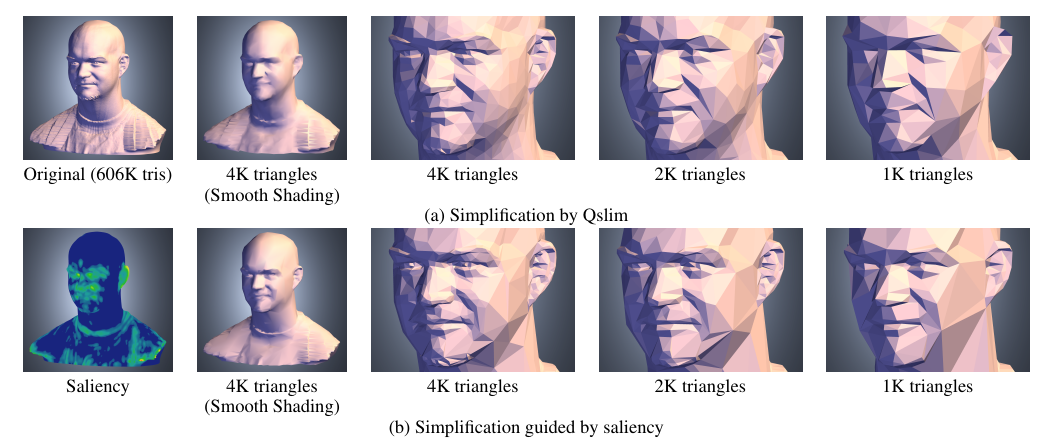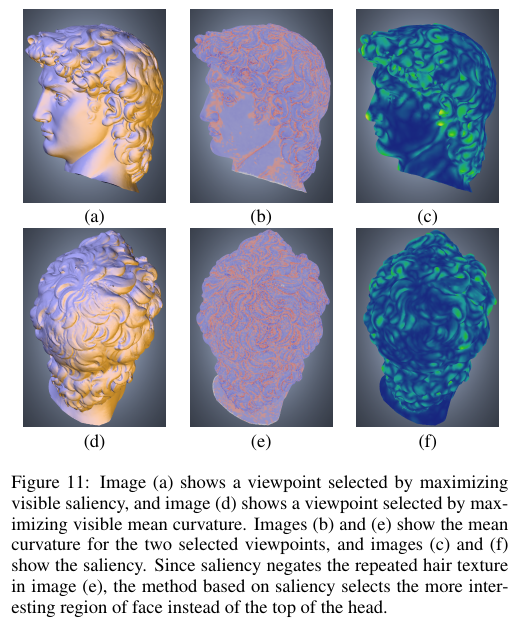# 算法

$G(\mathscr{C}(v), \sigma)=\frac{\sum_{x \in N(v, 2 \sigma)} \mathscr{C}(x) e^{-\|x-v\|^{2} / (2 \sigma^{2})}}{\sum_{x \in N(v, 2 \sigma)} e^{-\|x-v\|^{2} / (2 \sigma^{2})}}$

$\mathscr{S}(v)=|G(\mathscr{C}(v), \sigma)-G(\mathscr{C}(v), 2 \sigma)|$

$\mathscr{S}=\sum_{i} (M_i-\bar{m}_i)^{2} \mathscr{S}_{i}$

Saliency计算非常简单，到这里就结束了，所以下面是对重要性的两个简单应用。

# 应用：模型简化

### Saliency的改进

$\mathscr{W}(v)=A(\mathscr{S}(v), \alpha, \lambda)=\left\{\begin{array}{ll} \lambda \mathscr{S}(v) & \text { if } \mathscr{S}(v)>=\alpha \\ \mathscr{S}(v) & \text { if } \mathscr{S}(v)<\alpha \end{array}\right.$# 应用：视角选择

$U(v)=\Sigma_{x \in F(v)} \mathscr{S}(x)$©️2019 CSDN 皮肤主题: 书香水墨 设计师: CSDN官方博客